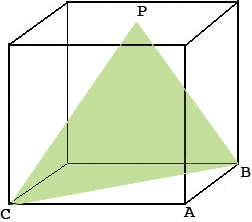SEARCH HOMEMath Central Quandaries & QueriesQuestion from Sohle: A cube has an edge of 4 cm. A triangle is formed by joining the middle point of one face of the cube to the ends of a diagonal of the opposite face . What will be the area of the triangle ?Hi,

Here is my diagram of what you described.$P$ is the midpoint of the top face and $BC$ is a diagonal of the opposite face. The triangle in question is green. Use Pythagoras theorem to find the base of the triangle. What is its height? What is its area?

Penny* Registered trade mark of Imperial Oil Limited. Used under license.Math Central is supported by the University of Regina and the Imperial Oil Foundation.